# youngsoft/TangramKit

TangramKit is a powerful iOS UI framework implemented by Swift. It integrates the functions with Android layout,iOS AutoLayout,SizeClass, HTML CSS float and flexbox and bootstrap. So you can use LinearLayout,RelativeLayout,FrameLayout,TableLayout,FlowLayout,FloatLayout,LayoutSizeClass to build your App 自动布局 UIView UITableView UICollectionView
1. Swift 99.6%
2. Other 0.4%oubaiquan Release 1.4.0
Latest commit 100faaa May 19, 2019
Type Name Latest commit message Commit time
Failed to load latest commit information.TangramKit.xcodeproj May 19, 2019TangramKit May 19, 2019TangramKitDemo May 19, 2019.gitignore Oct 27, 2016.swift-version Apr 25, 2019CHANGELOG.md May 19, 2019LICENSE Oct 27, 2016README.md May 6, 2018README.zh.md May 6, 2018TangramKit.podspec May 19, 2019## TangramKitTangramKit is a simple and easy Swift framework for iOS view layout. The name comes from Tangram of China which provides some simple functions to build a variety of complex interface. It integrates the functions including: Autolayout and SizeClass of iOS, five layout classes of Android, float and flex-box and bootstrap of HTML/CSS. The TangramKit's objective-C version are named: MyLayout

## Usage

• There is a container view S which width is 100 and height is wrap to all subviews height. there are four subviews A,B,C,D arranged from top to bottom.
• Subview A's left margin is 20% width of S, right margin is 30% width of S, height is equal to width of A.
• Subview B's left margin is 40, width is filled in to residual width of S,height is 40.
• Subview C's width is filled in to S, height is 40.
• Subview D's right margin is 20, width is 50% width of S, height is 40```    let S = TGLinearLayout(.vert)
S.tg_vspace = 10
S.tg_width.equal(100)
S.tg_height.equal(.wrap)
//you can use S.tg_size(width:100, height:.wrap) to instead

let A = UIView()
A.tg_left.equal(20%)
A.tg_right.equal(30%)
A.tg_height.equal(A.tg_width)

let B = UIView()
B.tg_left.equal(40)
B.tg_width.equal(.fill)
B.tg_height.equal(40)

let C = UIView()
C.tg_width.equal(.fill)
C.tg_height.equal(40)

let D = UIView()
D.tg_right.equal(20)
D.tg_width.equal(50%)
D.tg_height.equal(40)

```

TangramKit has override operators: ~=、>=、<=、+=、-=、*=、/= to implement equal、max、min、add、offset、multiply methods of `TGLayoutSize` and `TGLayoutPos` class, so you can instead to:

```let S = TGLinearLayout(.vert)
S.tg_vspace = 10
S.tg_width ~=100
S.tg_height ~=.wrap

let A = UIView()
A.tg_left ~=20%
A.tg_right ~=30%
A.tg_height ~=A.tg_width

let B = UIView()
B.tg_left ~=40
B.tg_width ~=.fill
B.tg_height ~=40

let C = UIView()
C.tg_width ~=.fill
C.tg_height ~=40

let D = UIView()
D.tg_right ~=20
D.tg_width ~=50%
D.tg_height ~=40
```

## Performance comparison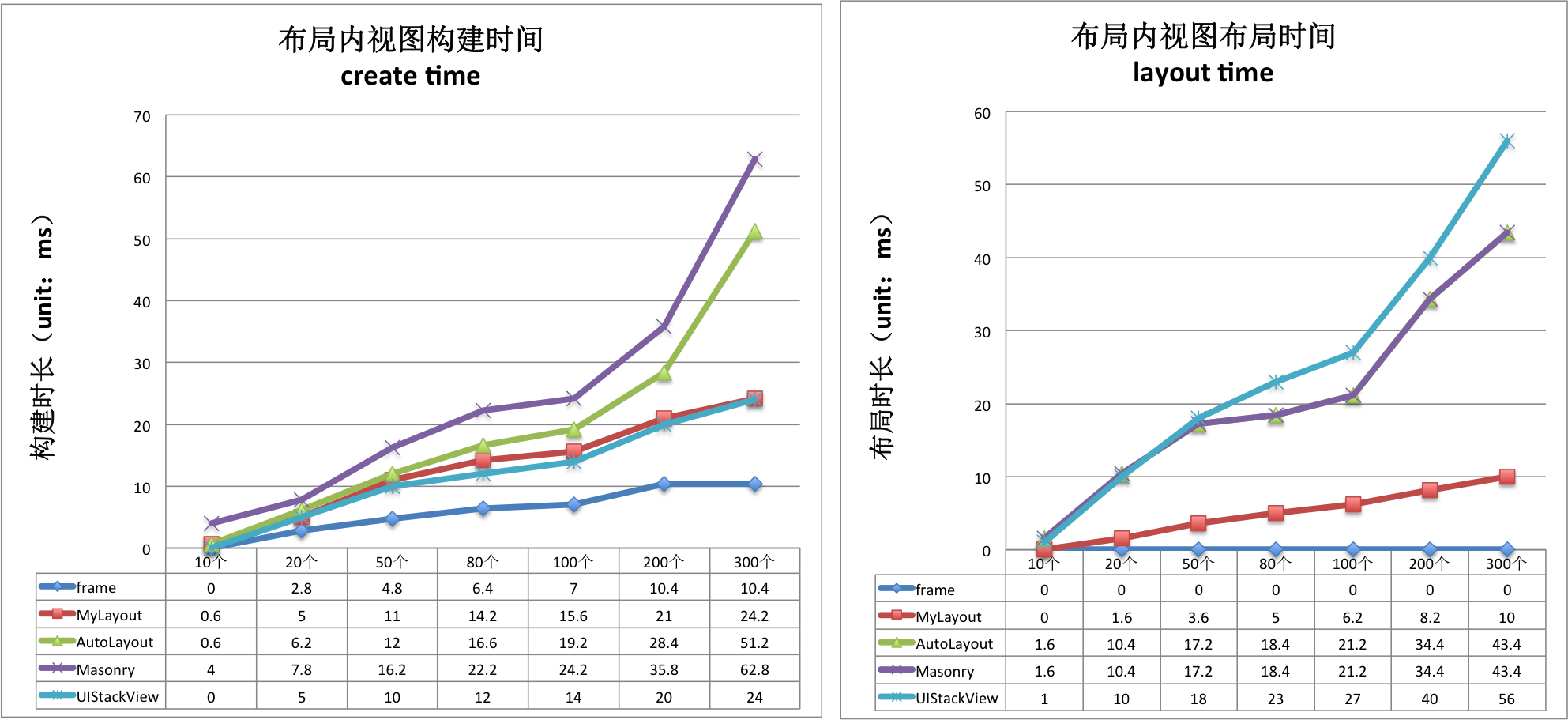create time(ms)/per subview Frame TangramKit AutoLayout Masonry UIStackView
TGLinearLayout 0.08 0.164 0.219 0.304 0.131
TGFrameLayout 0.05 0.149 0.209 0.273 0.131
TGRelativeLayout 0.079 0.182 0.116 0.359 0.131
TGFlowLayout 0.08 0.107 0.198 0.258 0.131
TGFloatLayout 0.044 0.148 0.203 0.250 0.131
layout time(ms)/per subview Frame TangramKit AutoLayout Masonry UIStackView
TGLinearLayout 0 0.049 0.269 0.269 0.272
TGFrameLayout 0 0.042 0.243 0.243 0.272
TGRelativeLayout 0 0.068 0.274 0.274 0.272
TGFlowLayout 0 0.036 0.279 0.279 0.272
TGFloatLayout 0 0.055 0.208 0.208 0.272

## Architecture### TGLayoutPos

`TGLayoutPos` is represent to the position of a view. UIView provides six extension variables:tg_left, tg_top, tg_bottom, tg_right, tg_centerX, tg_centerY to set view's margin or space distance between self and others.

### TGLayoutSize

`TGLayoutSize` is represent to the size of a view. UIView provides two extension variables:tg_width,tg_height to set view's width and height dimension. there are three special TGLayoutSize const object: `.wrap`, `.fill`, `.average` mean: wrap all subviews size, fill in to superview's residual size, average the superview's size.

### TGWeight

`TGWeight` is used to set relative position and dimension. TangramKit override operator % to easily construct a TGWeight object. e.g 20% is equal to TGWeight(20).

### TGLinearLayout

Is equivalent to: UIStackView of iOS and LinearLayout of Android.

Linear layout is a single line layout view that the subviews are arranged in sequence according to the added order（from top to bottom or from left to right). So the subviews' origin&size constraints are established by the added order. Subviews arranged in top-to-bottom order is called vertical linear layout view, and the subviews arranged in left-to-right order is called horizontal linear layout.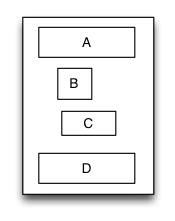```override func loadView() {

let S = TGLinearLayout(.vert)
S.tg_width.equal(120)
S.tg_height.equal(.wrap)
S.tg_vspace = 10

let A = UIView()
A.tg_left.equal(5)
A.tg_right.equal(5)
A.tg_width.equal(100)
A.tg_height.equal(40)

let B = UIView()
B.tg_left.equal(20)
B.tg_width.equal(40)
B.tg_height.equal(40)

let C = UIView()
C.tg_right.equal(40)
C.tg_width.equal(50)
C.tg_height.equal(40)

let D = UIView()
D.tg_left.equal(10)
D.tg_right.equal(10)
D.tg_width.equal(100)
D.tg_height.equal(40)

S.backgroundColor = .red
A.backgroundColor = .green
B.backgroundColor = .blue
C.backgroundColor = .orange
D.backgroundColor = .cyan
}
```

### TGRelativeLayout

Is equivalent to: AutoLayout of iOS and RelativeLayout of Android.

Relative layout is a layout view that the subviews layout and position through mutual constraints.The subviews in the relative layout are not depended to the adding order but layout and position by setting the subviews' constraints.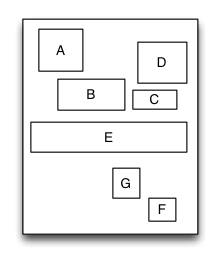```override func loadView() {

let S = TGRelativeLayout()
S.tg_width.equal(170).and().tg_height.equal(280)

let A = UIView()
A.tg_left.equal(20).and().tg_top.equal(20)
A.tg_width.equal(40).and().tg_height.equal(A.tg_width)

let B = UIView()
B.tg_left.equal(A.tg_centerX).and().tg_top.equal(A.tg_bottom).offset(10)
B.tg_width.equal(60).and().tg_height.equal(A.tg_height)

let C = UIView()
C.tg_left.equal(B.tg_right).offset(10)
C.tg_bottom.equal(B.tg_bottom)
C.tg_width.equal(40)
C.tg_height.equal(B.tg_height, multiple:0.5)

let D = UIView()
D.tg_bottom.equal(C.tg_top).offset(10)
D.tg_right.equal(15)
D.tg_height.equal(A.tg_height)
D.tg_width.equal(D.tg_height)

let E = UIView()
E.tg_centerY.equal(0)
E.tg_centerX.equal(0)
E.tg_height.equal(40)
//...F,G

S.backgroundColor = .red
A.backgroundColor = .green
B.backgroundColor = .blue
C.backgroundColor = .orange
D.backgroundColor = .cyan
E.backgroundColor = .magenta
}
```

### TGFrameLayout

Is equivalent to: FrameLayout of Android.

Frame layout is a layout view that the subviews can be overlapped and gravity in a special location of the superview.The subviews' layout position&size is not depended to the adding order and establish dependency constraint with the superview. Frame layout devided the vertical orientation to top,vertical center and bottom, while horizontal orientation is devided to left,horizontal center and right. Any of the subviews is just gravity in either vertical orientation or horizontal orientation.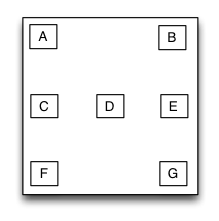``` override func loadView() {

let S = TGFrameLayout()
S.tg_width.equal(320)
S.tg_height.equal(500)

let A = UIView()
A.tg_width.equal(40)
A.tg_height.equal(40)

let B = UIView()
B.tg_width.equal(40)
B.tg_height.equal(40)
B.tg_right.equal(0)

let C = UIView()
C.tg_width.equal(40)
C.tg_height.equal(40)
C.tg_centerY.equal(0)

let D = UIView()
D.tg_width.equal(40)
D.tg_height.equal(40)
D.tg_centerY.equal(0)
D.tg_centerX.equal(0)

//..E，F,G

S.backgroundColor = .red
A.backgroundColor = .green
B.backgroundColor = .blue
C.backgroundColor = .orange
D.backgroundColor = .cyan
}
```

### TGTableLayout

Is equivalent to: TableLayout of Android and table of HTML.

Table layout is a layout view that the subviews are multi-row&col arranged like a table. First you must create a rowview and add it to the table layout, then add the subview to the rowview. If the rowviews arranged in top-to-bottom order,the tableview is caled vertical table layout,in which the subviews are arranged from left to right; If the rowviews arranged in in left-to-right order,the tableview is caled horizontal table layout,in which the subviews are arranged from top to bottom.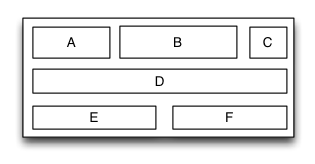```   override func loadView() {

let S = TGTableLayout(.vert)
S.tg_height.equal(.wrap)
S.tg_width.equal(.wrap)
S.tg_vspace = 10
S.tg_hspace = 10

let A = UIView()
A.tg_width.equal(50)
A.tg_height.equal(40)

let B = UIView()
B.tg_width.equal(100)
B.tg_height.equal(40)

let C = UIView()
C.tg_width.equal(30)
C.tg_height.equal(40)

let D = UIView()
D.tg_width.equal(200)
D.tg_height.equal(40)

//...E,F

S.backgroundColor = .red
A.backgroundColor = .green
B.backgroundColor = .blue
C.backgroundColor = .orange
D.backgroundColor = .cyan
}

```

### TGFlowLayout

Is equivalent to: flexbox of CSS3.

Flow layout is a layout view presents in multi-line that the subviews are arranged in sequence according to the added order, and when meeting with a arranging constraint it will start a new line and rearrange. The constrains mentioned here includes count constraints and size constraints. The orientation of the new line would be vertical and horizontal, so the flow layout is divided into: count constraints vertical flow layout, size constraints vertical flow layout, count constraints horizontal flow layout, size constraints horizontal flow layout. Flow layout often used in the scenes that the subviews is arranged regularly, it can be substitutive of UICollectionView to some extent. the TGFlowLayout is almost implement the flex-box function of the HTML/CSS.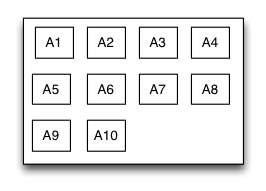```   override func loadView() {

let S = TGFlowLayout(.vert,arrangedCount:4)
S.tg_height.equal(.wrap)
S.tg_width.equal(300)
S.tg_gravity = TGGravity.horz.fill
S.tg_space = 10

for _ in 0 ..< 10
{
let A = UIView()
A.tg_height.equal(A.tg_width)

A.backgroundColor = .green
}

S.backgroundColor = .red
}

```

### TGFloatLayout

Is equivalent to: float of CSS.

Float layout is a layout view that the subviews are floating gravity in the given orientations, when the size is not enough to be hold, it will automatically find the best location to gravity. float layout's conception is reference from the HTML/CSS's floating positioning technology, so the float layout can be designed in implementing irregular layout. According to the different orientation of the floating, float layout can be divided into left-right float layout and up-down float layout.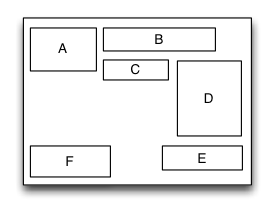```     override func loadView() {

let S = TGFloatLayout(.vert)
S.tg_height.equal(.wrap)
S.tg_width.equal(300)
S.tg_space = 10

let A = UIView()
A.tg_width.equal(80)
A.tg_height.equal(70)

let B = UIView()
B.tg_width.equal(150)
B.tg_height.equal(40)

let C = UIView()
C.tg_width.equal(70)
C.tg_height.equal(40)

let D = UIView()
D.tg_width.equal(100)
D.tg_height.equal(140)

let E = UIView()
E.tg_width.equal(150)
E.tg_height.equal(40)
E.tg_reverseFloat = true

let F = UIView()
F.tg_width.equal(120)
F.tg_height.equal(60)

S.backgroundColor = .red
A.backgroundColor = .green
B.backgroundColor = .blue
C.backgroundColor = .orange
D.backgroundColor = .black
E.backgroundColor = .magenta
F.backgroundColor = .white
}
```

### TGPathLayout

Is unique characteristic layout view of iOS.

Path layout is a layout view that the subviews are according to a specified path curve to layout. You must provide a type of Functional equation，a coordinate and a type of distance setting to create a Path Curve than all subview are equidistance layout in the Path layout. path layout usually used to create some irregular and gorgeous UI layout.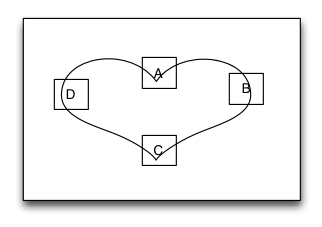Sample code:

```override func loadView()
{

let S = TGPathLayout()
S.tg_width.equal(320)
S.tg_height.equal(320)
S.tg_coordinateSetting.isReverse = true
S.tg_coordinateSetting.origin = CGPoint(x: 0.5, y: 0.2)
S.tg_polarEquation = { 80 * (1 + cos(CGFloat(\$0))) } // r = a *(1 + cos(𝛉))

for _ in 0 ..< 4
{
let A = UIView()
A.tg_size(width:40,height:40)

A.backgroundColor = .green
}

S.backgroundColor = .red
}

```

### TGViewSizeClass

Is equivalent to: Size Classes of iOS.

TangramKit provided support to SizeClass in order to fit the different screen sizes of devices. You can combinate the SizeClass with any of the 6 kinds of layout views mentioned above to perfect fit the UI of all equipments.

```    public func tg_fetchSizeClass(with type:TGSizeClassType, from srcType:TGSizeClassType! = nil) ->TGViewSizeClass

```
```//all device
let rootLayout = TGLinearLayout(.vert)
rootLayout.tg_padding = UIEdgeInsetsMake(10, 10, 10, 10);
rootLayout.tg_vspace = 10
rootLayout.tg_hspace = 10

//iPhone landscape orientation.
let lsc = rootLayout.tg_fetchSizeClass(with: .comb(.any, .compact, nil), from:.default) as! TGLinearLayoutViewSizeClass
lsc.tg_orientation = .horz
```

## Communication

• If you need help, use Stack Overflow or Baidu. (Tag 'TangramKit')
• If you'd like to contact me, use qq:156355113 or weibo:欧阳大哥 or email:obq0387_cn@sina.com
• If you found a bug, and can provide steps to reliably reproduce it, open an issue.
• If you have a feature request, open an issue.
• If you want to contribute, submit a pull request.

## Installation

TangramKit supports multiple methods for installing the library in a project.

1. Copy `TangramKit` folder from the demo project to your project

### Installation with CocoaPods

CocoaPods is a dependency manager for Objective-C, which automates and simplifies the process of using 3rd-party libraries like TangramKit in your projects. You can install it with the following command:

`\$ gem install cocoapods`

To integrate TangramKit into your Xcode project using CocoaPods, specify it in your Podfile:

``````source 'https://github.com/CocoaPods/Specs.git'
platform :ios, '8.0'

pod 'TangramKit'
``````

Then, run the following command:

`\$ pod install`

### Use Carthage

1. Create a `Cartfile` file.

``````github "youngsoft/TangramKit"
``````
2. Run `carthage update`.

3. On your application targets’ “General” settings tab, in the “Linked Frameworks and Libraries” section, drag and drop `TangramKit` framework from the Carthage/Build folder on disk.

4. On your application targets’ “Build Phases” settings tab, click the “+” icon and choose “New Run Script Phase”. Create a Run Script in which you specify your shell (ex: bin/sh), add the following contents to the script area below the shell:

``````/usr/local/bin/carthage copy-frameworks
``````

and add the path under “Input Files”, e.g.:

``````\$(SRCROOT)/Carthage/Build/iOS/TangramKit.framework
``````

## FAQ

• If you use TangramKit runtime cause 100% CPU usage said appeared constraint conflict, please check the subview's constraint set.